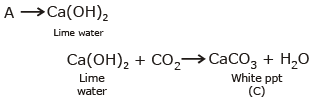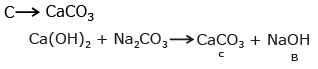Courses

# Test: s-Block Elements- 2

## 30 Questions MCQ Test Chemistry for JEE | Test: s-Block Elements- 2

Description
This mock test of Test: s-Block Elements- 2 for Class 11 helps you for every Class 11 entrance exam. This contains 30 Multiple Choice Questions for Class 11 Test: s-Block Elements- 2 (mcq) to study with solutions a complete question bank. The solved questions answers in this Test: s-Block Elements- 2 quiz give you a good mix of easy questions and tough questions. Class 11 students definitely take this Test: s-Block Elements- 2 exercise for a better result in the exam. You can find other Test: s-Block Elements- 2 extra questions, long questions & short questions for Class 11 on EduRev as well by searching above.
QUESTION: 1

### Cs+ ions impart violet to Bunsen flame. This is due to the fact that the emitted radiations are of

Solution:

Emmited wavelength lies in visible region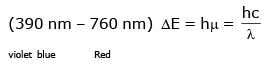Blue has high frequency low wavelength -high energy

QUESTION: 2

### When sodium is dissolved in liquid ammonia, a solution of deep blue colour is obtained. The colour of the solution is due to

Solution:

Be2+ →  Al3+ + Be2+ is diagonaly similar with Al3+ & Al3+ is amphoteric in nature.

QUESTION: 3

### An alkaline earth metal (M) gives a salt with chlorine, which is soluble in water at room temperature. It also forms an insoluble sulphate whose mixture with a sulphide of a transition metal is called `lithopone'-a white pigment. Metal M is-

Solution:

Lithopane is (BaSO4 + ZnS)
white pigment
BaSO4 is insoluble in water

QUESTION: 4

The reaction of an element A with water produces combustible gas B and an aqueous solution of C. When another substance D reacts with this solution C also produces the same gas B.D also produces the same gas even on reaction with dilute H2SO4 at room temperature. Element A imparts golden yellow colour to Bunsen flame. Then A,B,C and D may be identified as

Solution: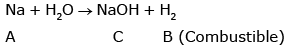Zn + NaOH → Na2ZnO2 + H2
Amphoteric
Nz + dil H2SO4 → ZnSO4 + H2

QUESTION: 5

The hydroxide of alkaline earth metal, which has the lowest value of solubility product (Ksp) at normal temperature (25°C) is-

Solution:

Be(OH)2 down the group solubility increase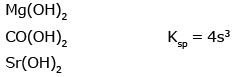QUESTION: 6

(Yellow ppt) T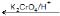X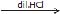Y. (Yellow ppt) + Z­ (pungent smellinggas)

If X gives green flame test. Then, X is :

Solution:

Green flame is given by Ba2+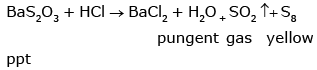BaS2O3+K2CrO4 → BaCrO4+K2S2O3   yellow ppt

QUESTION: 7

Which of the following carbonate of alkali metals has the least thermal stability ?

Solution:

T.S ∞ Ionic character (for polyatomic anion)
Li2CO3 is least ionic or most covalent

QUESTION: 8

The `milk of magnesia' used as an antacid is chemicaly -

Solution:

Mg(OH)2
Base or Antacid which distroys and as per neutralisation reaction

QUESTION: 9

The solubility of metal halides depends on their nature, lattice enthalpy and hydration enthalpy of the individual ions. Amongst fluorides of alkali metals, the lowest solubility of LiF in water is due to

Solution:

K2O,    KO2,  K2O2
Rb2O,   RbO2,   Rb2O2
Na, Li from normal oxide

QUESTION: 10

The pair of compounds, which cannot exist together in a solution is-

Solution:

NaHCO3 + NaOH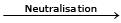Na2CO3 + H2O

Acidic  Base hydrogen

QUESTION: 11

The formula of soda ash is

Solution:

Soda ash is Na2CO3.

QUESTION: 12

The hydration energy of Mg2+ is -?

Solution:

Hydration energy ∞ polarising power
Na+ < Mg2+ < Mg3+ < Be3+ < Al3+

QUESTION: 13

The golden yellow colour associated with NaCl to Bunsen flame can be explained on the basis of -

Solution:

In frame test thermal excitation deexcitation takes place in cation with low Ionisation potential

QUESTION: 14

Solution of sodium metal in liquid ammonia is a strong reducing agent due to presence of -?

Solution: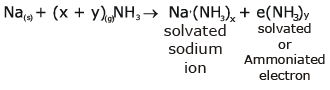QUESTION: 15

The order of solubility of lithium halides in non polar solvents follows the order.

Solution:

As the size of the anion increases fromto, the covalent character increase and hence the solubility in non-polar solvent increases.QUESTION: 16

The salt which finds uses in qualitative inorganic analysis is -?

Solution:

Microcosinic salt is used in microcosmicbed test

QUESTION: 17

Fire extinguishers contain -?

Solution:

NaHCO3 + H2SO4 →  Na2SO4 + H2O + CO2
extingusher fire

QUESTION: 18

CsBr3 contains -?

Solution:

Cs is highly electropositive & forms ionic bond with Cs+ cation.

QUESTION: 19

Alkali metals reacts with water vigorously to form hydroxides and dihydrogen. Which of the following alkali metals reacts with water least vigorously?

Solution:

Na + Al2O3 → Na2O + Al (Position in electrochemical series)
Na2O + CO2 → Na2CO3

QUESTION: 20

The correct order of second ionisation potentials (IP) of Ca, Ba and K is -

Solution:

K [Ar] 4s1 →  second ionisation is extremely difficult.
Ca [Ar] 4s2
Ba  [Xe] 5S2

QUESTION: 21

EDTA is used in the estimation of - ?

Solution: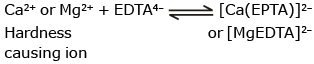QUESTION: 22

aq. NaOH + P4 (white) → PH3 + X; compound X is

Solution: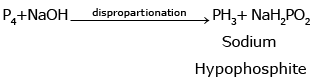QUESTION: 23

The correct order of solubility is -?

Solution:

Evident from Fajan rule

QUESTION: 24

The complex formation tendency of alkaline earth metals decreases down the group because -

Solution:

Down the group Zeff decrease & complex farmation tendeny decrease

QUESTION: 25

The alkaline earth metals, which do not impart any colour to Bunsen flame are -

Solution:

Be & Mg due to small size does not impart colour to the flame as excitation & deexcitation is not possible.

QUESTION: 26

The alkali metals are low melting. Which of the following alkali metal is expected to melt if the room temperature rises to 30°C?

Solution:

(d) Among alkali metals, melting point decreases as the strength of metallic bonding decreases with increasing size of the atom. Thus, Cs has the lowest melting point (28.5°C) and will melt at 30°C.

QUESTION: 27

A metal M readily forms water soluble sulphate, and water insoluble hydroxide M(OH)2. Its oxide MO is amphoteric, hard and having high melting point. The alkaline earth metal M must be -

Solution:

BeO is amphoteric due to its diogional similarly with Al

QUESTION: 28

When K2O is added to water, the solution becomes basic in nature because it contains a significant concentration of -

Solution:

K2O + H2O → KOH

QUESTION: 29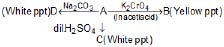If A is the metallic salt, then the white ppt. of D must be of -?

Solution: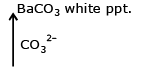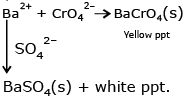QUESTION: 30

(Mily Cloud)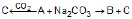The chemical formulae of A and B are -?

Solution: